##### Pre-Calculus: 1001 Practice Problems For Dummies (+ Free Online Practice)A system of equations is a collection of two or more equations involving two or more variables. If the number of equations is equal to the number of different variables, then you may be able to find a unique solution that’s common to all the equations.

Having the correct number of variables isn’t a guarantee that you’ll have that solution, and it’s not terrible if a unique solution doesn’t exist; sometimes you just write a rule to represent the many solutions shared by the equations in the collection.

You’ll work on solving systems of equations in the following ways:

• Using substitution to solve linear and nonlinear systems of equations

• Applying the elimination method when solving systems of linear equations

• Writing a rule for multiple solutions of systems of equations

• Creating partial fractions using fraction decomposition

• Writing coefficient matrices and constant matrices to use in matrix solutions of systems

• Determining matrix inverses to use in solving systems of linear equations

When you’re working with systems of equations, some challenges will include

• Recognizing that the answer may be no solution

• Distributing correctly when using substitution for solving systems

• Performing matrix operations correctly when doing row reductions and eliminating terms

• Writing solutions from resulting variable matrices

## Practice problems

1. Solve each system of equations. Write the solution as an ordered triple, (x, y, z).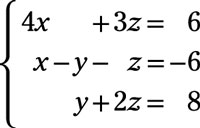Eliminate x in the first equation. To do so, multiply the second equation (xyz = –6) by –4 and add it to the first equation: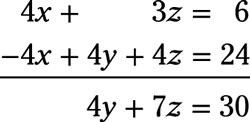Now use this new equation and the original third equation to eliminate y. Multiply the third equation (y + 2z = 8) by –4 and add it to the new equation: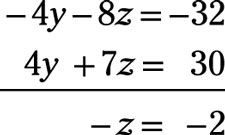Multiply each side of the equation by –1 to get z = 2.

Substitute 2 for z in the original third equation to solve for y: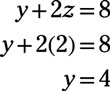To solve for x, substitute 2 for z in the original first equation: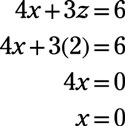In (x, y, z) form, the answer is (0, 4, 2).

2. Solve the system of equations. Write the solution as (x, y, z, w):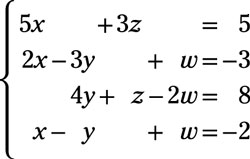Start by eliminating the w term. Multiply the second equation (2x – 3y + w = –3) by 2 and add it to the third equation: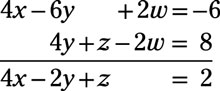Next, multiply the fourth equation (xy + w = –2) by 2 and add it to the third equation: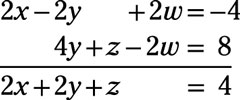The new system of equations, without the y term, consists of these two new equations and the original first equation: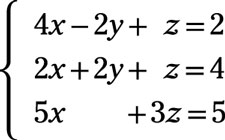The next step involves eliminating the y term. Add the first two equations of the new system together: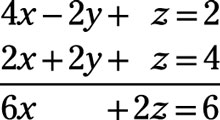Each term in the new equation is divisible by 2, giving you 3x + z = 3. Multiply the terms in this equation by –3 and add it to the last equation in the new system: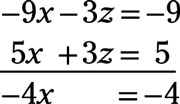Dividing by –4, you have x = 1. Now back-solve to find the values of the rest of the variables: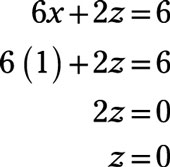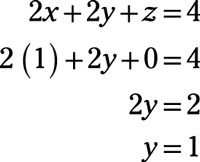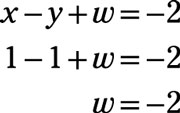In (x, y, z, w) form, the answer is (1, 1, 0, –2).